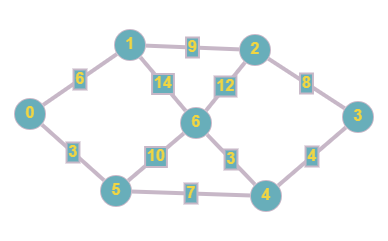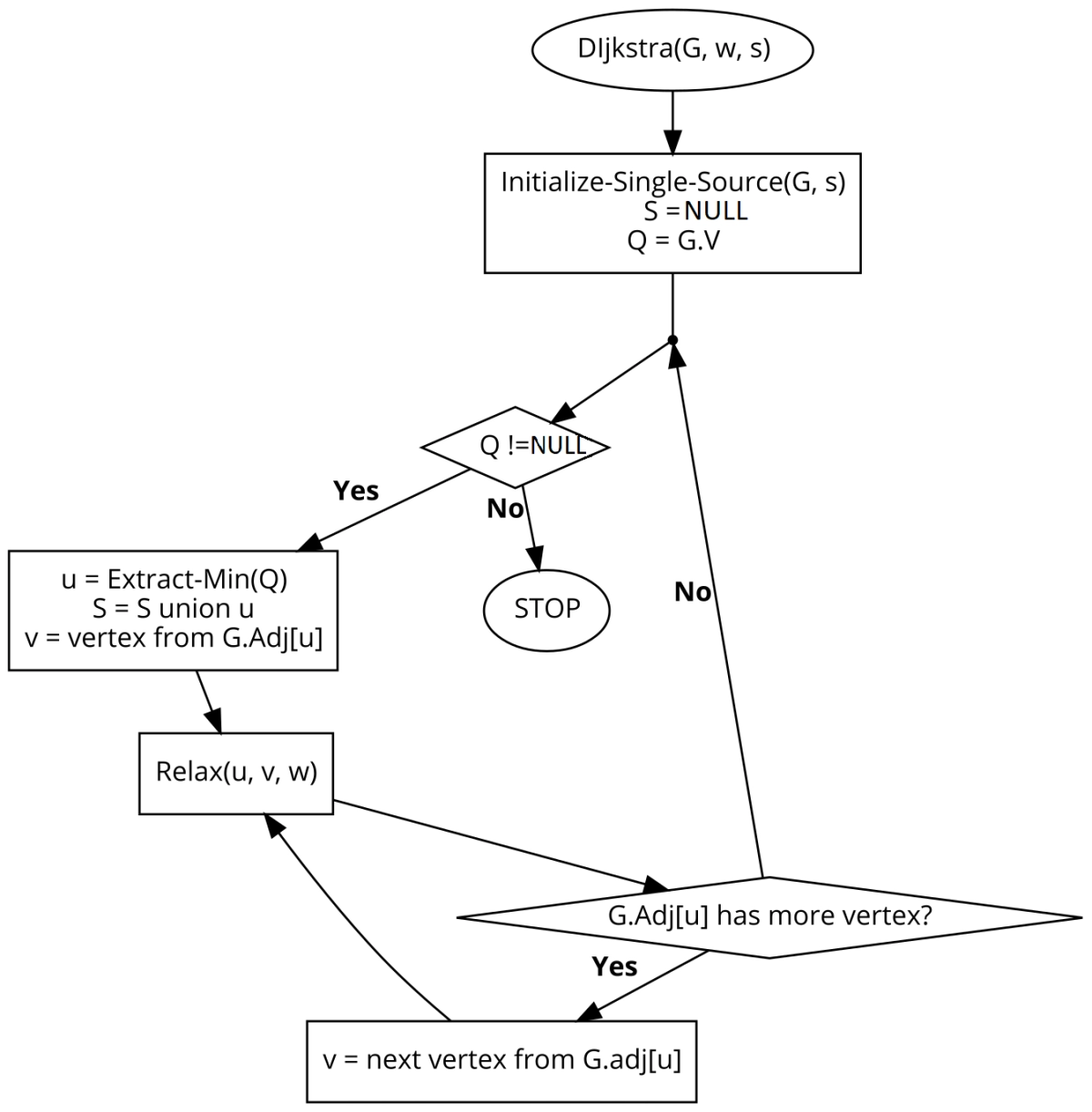New update is available. Click here to update.
Last Updated: Apr 29, 2023
Easy

# Dijkstra's Algorithm0 upvotes

## Introduction

This article discusses Dijkstra’s Algorithm with the help of various examples and code pieces. Dijkstra’s algorithm is one of the essential algorithms that a programmer must be aware of to succeed. The algorithm is straightforward to understand and has a vast horizon of applications.

Some of its famous uses are:

• It is used in DNA mapping
• It is used in Google Maps

Dijkstra’s Algorithm uses the concepts of Greedy Algorithms. Readers with no prior knowledge of greedy algorithms are requested to follow the link to know more.

## Basic Idea

Given a graph G, with some vertices and edge weights, find the minimum cost of travelling from the source vertex to each vertex. The graph can be undirected or directed.

The output displays the minimum cost when travelling from the source vertex to the destination vertex.

## Example and Explanation

Let us take an example.The above-shown graph is an undirected graph with 7 vertices and some edge weights. The input array for this graph will be:

int inputGraph[V][V] = {    { 0, 6, 0, 0, 0, 3, 0},
{ 6, 0, 9, 0, 0, 0, 0},
{ 0, 9, 0, 8, 0, 0, 12},
{ 0, 0, 8, 4, 0, 0, 0},
{ 0, 0, 0, 4, 0, 7, 3},
{ 3, 0, 0, 0, 7, 0, 10},
{ 0, 14, 12, 0, 3, 10, 0}
};

The output from the input graph is:

Vertex   Distance from Source
0               0
1               6
2               15
3               14
4               10
5               3
6               13

• We calculate the minimum cost that occurred while travelling from the source vertex (0 in our case) to all the other vertices.

• For vertex 0, we are travelling from 0 to 0. Thus the minimum cost is 0.

• For vertex 1, our source vertex is 0, and the destination vertex is 1. Thus the minimum cost is 6.

• For vertex 5, our source vertex is 0, and the destination vertex is 5. The minimum cost is 3.

• A case where we have to choose between two paths is at vertex 6. The minimum cost till vertex 1 is six, and till vertex 5 is six. To travel to vertex 6, we have two routes- one via vertex 1 and another via vertex 5.
• If we take the path 0 -> 1 -> 6, then our cost comes out to be 6+14=20.
• If we take the path 0 -> 5 -> 6, then our cost comes out to be 3+10=13.
• So, we choose the minimum of the two and set the minimum cost till vertex 6 from vertex 0 to 13.

• We continue this process until all the vertices have been travelled. Once all the vertices are travelled, we print the min-cost in the given manner.

## Approach 1

• First, we create two 1D arrays- dist and sptSet. The dist array will contain the minimum cost from the source to that vertex. The sptSet will be a boolean array that will store whether we have found the minimum cost to a vertex or not.

• Initialize the 0th index of dist as 0 and the rest as INFINITY.
• In our implementation, we have selected vertex 0 as our single source vertex.
• All the distances will be calculated from 0 to that vertex.
• After initialization of dist array, it will look like this- {0, INF, INF, INF, INF, INF}, where INF is INFINITY.
• Since the minimum cost to travel from vertex 0 to itself is 0, we initialize it as zero and others as INFINITY.

• Now, for every vertex, find the distance u using the minDist() function.

• Inside the minDist() function, we take two variables- min and min_idx. The min value is initialized with INF and will store and return the minimum distance from one untravelled vertex to another connected vertex.

• If this distance u is less than the previously stored distance, update the dist array with u.

• Do this for all the vertices. Once all the vertices have been traversed, the dist array will contain the minimum distance.

### Flowchart### Pseudocode

Dijkstra (G, S)
For each vertex v in graph G
D[S] = 0
D[V] = INF

Initialize visited vertices S in graph G
S = null
Initialize Queue Q as a set of all nodes in graph G
Q = all vertices v

while Q is not empty
u = mindist(Graph G, distance d)
Visited[S] = S + u
for each vertex V in neighbour[u]:
if d[V] > d[u] + w(u,v)
d[v] = d[u] + w(u,v)

return d

## C++ implementation of Approach 1

#include <iostream>
using namespace std;
#define V 7
#define INF 99999

int minDist(int dist[], bool sptSet[])
{
int min = INF, min_idx;

for (int v = 0; v < V; v++)
if (sptSet[v] == false && dist[v] <= min)
min = dist[v], min_idx = v;

return min_idx;
}

void printDist(int dist[])
{
cout <<"Vertex \t Distance from Source" << endl;
for (int i = 0; i < V; i++)
cout  << i << " \t\t"<<dist[i]<< endl;
}

void dijkstra(int graph[V][V], int src)
{
int dist[V];

bool sptSet[V];

for (int i = 0; i < V; i++){
dist[i] = INF;
sptSet[i] = false;
}

dist[src] = 0;

for (int i = 0; i < V - 1; i++) {

int u = minDist(dist, sptSet);

sptSet[u] = true;

for (int v = 0; v < V; v++){
if (!sptSet[v] && graph[u][v] && dist[u] != INF && dist[u] + graph[u][v] < dist[v])
dist[v] = dist[u] + graph[u][v];
}
}
printDist(dist);
}

int main()
{
int inputGraph[V][V] = {    { 0, 6, 0, 0, 0, 3, 0},
{ 6, 0, 9, 0, 0, 0, 0},
{ 0, 9, 0, 8, 0, 0, 12},
{ 0, 0, 8, 4, 0, 0, 0},
{ 0, 0, 0, 4, 0, 7, 3},
{ 3, 0, 0, 0, 7, 0, 10},
{ 0, 14, 12, 0, 3, 10, 0}
};

dijkstra(inputGraph, 0);
return 0;
}

Output

Vertex   Distance from Source
0               0
1               6
2               15
3               14
4               10
5               3
6               13

## Complexities

### Time Complexity

In approach 1, we traverse V columns for each V row. Thus, the time complexity is,

T(n) = O(V2),

where V is the number of vertices.

### Space Complexity

In approach 1, we are maintaining 1D two arrays. One to store the minimum dist, and another to store which vertices’ minimum cost has been found. Thus,

Space Complexity = O(V+V),

where V is the number of vertices.

After going through the introduction, example and solution, you must watch this video to understand better.

## Approach 2

The above solution might look easy to comprehend, but it has one flaw. Its time complexity is O(V2) which is too much. To reduce the time complexity of the above implementation, we use the concepts of the priority queue which reduces the time complexity to O(E log V)

We use a Binary Heap to implement our priority queue.

The step-by-step Java implementation of Approach 2 is as follows:

• Declare a min-heap array (say minHeap) which will store the minimum distance from the source vertex to the destination vertex. The size of the minHeap is V (number of vertexes).

• Initialize the 0th index to 0, and from 1 to V to infinity. We do so because we want our source vertex to be 0.

•  Now create a priority queue that will store all the visited vertices.

• Iterate while our priority queue is not empty.
• Now traverse each node of our input graph to find the shortest path from the source vertex to each vertex.

• If the traversal cost of the current path is less than the traversal cost stored in the minHeap, update the minHeap value with the current lower value.

• Add the new path cost of that vertex to the priority queue.

• Return the minHeap array which stores the minimum path cost from source to each vertex.

## Java Implementation of Approach 2

import java.util.ArrayList;
import java.util.PriorityQueue;

public class PQDijkstra {

static class AListNode {
int vertex, weight;

AListNode(int vertex, int weight)
{
this.vertex = vertex;
this.weight = weight;
}
int getVertex() { return vertex; }
int getWeight() { return weight; }
}

public static int[] dijkstra(int V, ArrayList<ArrayList<AListNode>> inpGraph, int source)
{
int[] minHeap = new int[V];

for (int i = 1; i < V; i++) {
minHeap[i] = Integer.MAX_VALUE;
}

minHeap = 0;

PriorityQueue<AListNode> pq = new PriorityQueue<>((v1, v2) -> v1.getWeight() - v2.getWeight());

while (pq.size() > 0) {
AListNode current = pq.poll();

for (AListNode n : inpGraph.get(current.getVertex())) {

if (minHeap[current.getVertex()] + n.getWeight() < minHeap[n.getVertex()]) {
minHeap[n.getVertex()] = n.getWeight() + minHeap[current.getVertex()];
}
}
}

return minHeap;
}

public static void main(String[] args)
{
int V = 7;
ArrayList<ArrayList<AListNode> > inpGraph = new ArrayList<>();

for (int i = 0; i < V; i++) {
}
int source = 0;

int[] retDist = dijkstra(V, inpGraph, source);

System.out.println("Vertex  " + "  Distance from Source");
for (int i = 0; i < V; i++) {
System.out.println(i + "             " + retDist[i]);
}
}
}

OUTPUT

Vertex    Distance from Source
0             0
1             6
2             15
3             14
4             10
5             3
6             13


## Complexities

### Time Complexity

In approach 2, we implement min-heap using a priority queue. This drastically reduces our time from O(V2) to O(E log V). Thus time complexity is,

T(N) = O(E log V),

where E is the number of edges and V is the number of Vertices.

### Space Complexity

In approach 2, we create a min-heap to store the minimum distance from the source vertex to each vertex. Thus,

Space Complexity = O(V),

where V is the number of vertices.

1. Can the Dijkstra Algorithm handle negative edge weights?
Dijkstra cannot handle the negative edges properly. In a few cases, it may yield the right solution, but it will fail most of the time. To properly handle negative edges, we use the Bellman-Ford algorithm.

2. What is the purpose of the Dijkstra Algorithm?
Dijkstra's algorithm solves the shortest-path problem for any weighted, directed graph with non-negative weights. It can handle graphs consisting of cycles, but negative weights will cause this algorithm to produce incorrect results.

## Key Takeaways

To summarize the article, we had a thorough discussion on Dijkstra’s Algorithm. We learned about the basic idea of the algorithm, an example with its explanation, a suitable approach, and the solution code. We also discussed the time and space complexities along with a few FAQs.

Recommended Problems:

Want to ace the coding rounds of big tech companies? Try our Attempt Unlimited Online Mock Test Series to start your preparation.

Learn various topics from Web Technologies, Programming Fundamentals, Data Structure, and Algorithms from our Library

Happy Coding!

Codekaze-June23 India's Biggest Tech Hiring Challenge is LIVE!Go on top